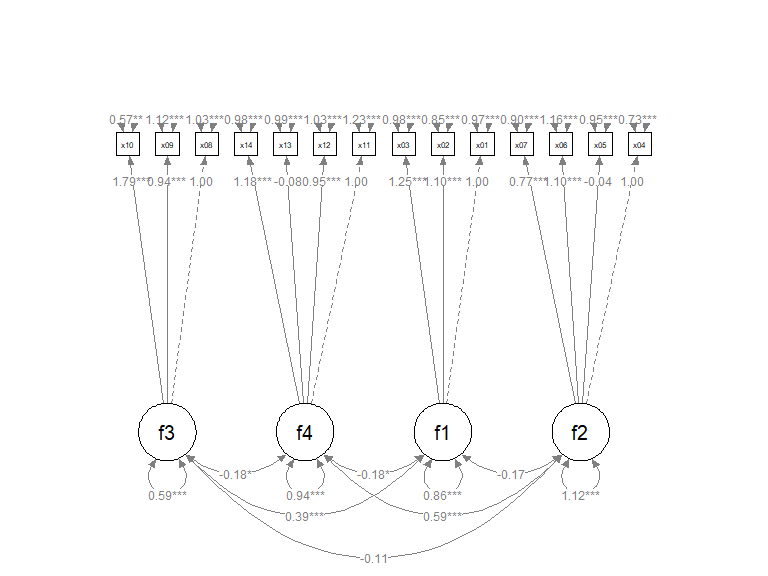# Introduction

The semptools package contains functions that post-process an output from semPlot::semPaths(), to help users to customize the appearance of the graphs generated by semPlot::semPaths(). For the introduction to functions for doing very specific tasks, such as moving the parameter estimate of a path or rotating the residual of a variable, please refer to vignette("semptools"). The present guide focuses on how to use set_cfa_layout() to configure various aspects of an semPaths graph generated for a typical confirmatory factor analysis (CFA) model.

# The Initial semPaths Graph

Let us consider a CFA model. We will use cfa_example, a sample CFA dataset from semptools with 14 variables for illustration.

library(semptools)
#>      x01    x02    x03    x04    x05    x06    x07   x08   x09    x10    x11
#> 1  1.159  1.271  1.451 -0.691 -0.015 -0.212 -0.336 1.559 0.870  1.115 -1.251
#> 2  0.059 -0.496 -0.585 -1.800 -0.555  0.012  1.208 0.551 0.055 -0.365 -0.142
#> 3 -0.737  2.933  1.625  0.642 -1.218 -0.155 -0.861 0.862 0.738  2.443 -0.628
#>     x12    x13    x14
#> 1 0.253  0.663 -1.049
#> 2 0.110 -0.207 -0.226
#> 3 1.604 -1.688  0.395

This is the CFA model to be fitted:

mod <-
'f1 =~ x01 + x02 + x03
f2 =~ x04 + x05 + x06 + x07
f3 =~ x08 + x09 + x10
f4 =~ x11 + x12 + x13 + x14
'

Fitting the model by lavaan::cfa()

library(lavaan)
fit <- lavaan::cfa(mod, cfa_example)

This is the plot from semPlot::semPaths():

library(semPlot)
p <- semPaths(fit, whatLabels="est",
sizeMan = 3.25,
node.width = 1,
edge.label.cex = .75,
style = "ram",
mar = c(10, 5, 10, 5))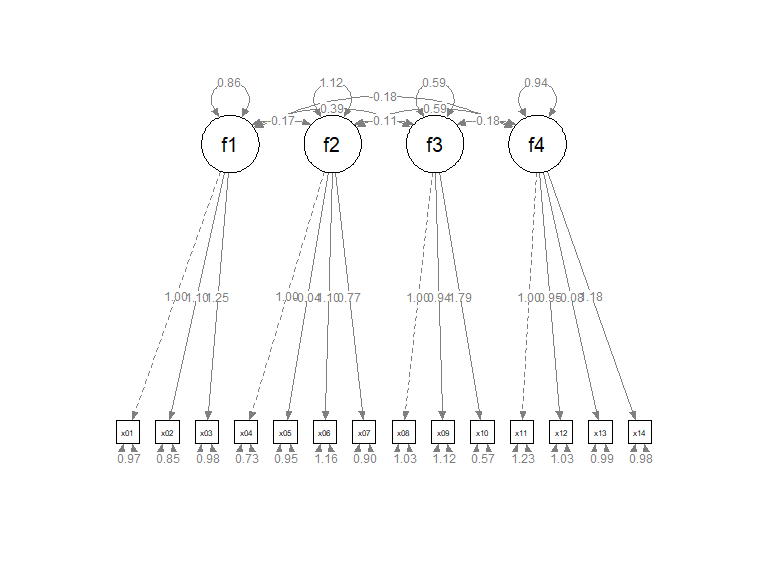The default layout is sufficient to have a quick examination of the results. We will see how set_cfa_layout() can be used to do the following tasks to post-process the graph:

• Change the order of the indicators.

• Change the order of the factors.

• Change the curvature of the inter-factor covariances.

• Rotate the graph.

# Order the Indicators and Factors

Suppose we want to do this:

• Order the factors this way, from the left to the right:

• f2, f1, f4, f3
• Order the indicators this way, from the left to the right:

• x04, x05, x06, x07, x01, x02, x03, x11, x12, x13, x14, x08, x09, x10
• We would like to place the factors this way:

• f2 above the center of x04, x05, x06, and x07.

• f1 above the center of x01, x02, and x03.

• f4 above the center of x11, x12, x13, and x14.

• f3 above the center of x08, x09, and x10.

To do this, we create two vectors, one for the argument indicator_order and the other for the argument indicator_factor.

• indicator_order is a string vector with length equal to the number of indicators, with the desired order. In this example, it will be like this:
indicator_order  <- c("x04", "x05", "x06", "x07",
"x01", "x02", "x03",
"x11", "x12", "x13", "x14",
"x08", "x09", "x10")
• indicator_factor is a string vector with length equal to the number of indicators. The elements are the names of the latent factors, denoting which indicators will be used to compute the mean positions to place the latent factors:
indicator_factor <- c( "f2",  "f2",  "f2",  "f2",
"f1",  "f1",  "f1",
"f4",  "f4",  "f4",  "f4",
"f3",  "f3",  "f3")

The set_cfa_layout() function needs at least three arguments:

• semPaths_plot: The semPaths plot.

• indicator_order: The vector for the order of indicators.

• indicator_factor: The vector for the placement of the latent factors.

They do not have to be named if they are in this order.

We now use set_cfa_layout() to post-process the graph:

p2 <- set_cfa_layout(p,
indicator_order,
indicator_factor)
plot(p2)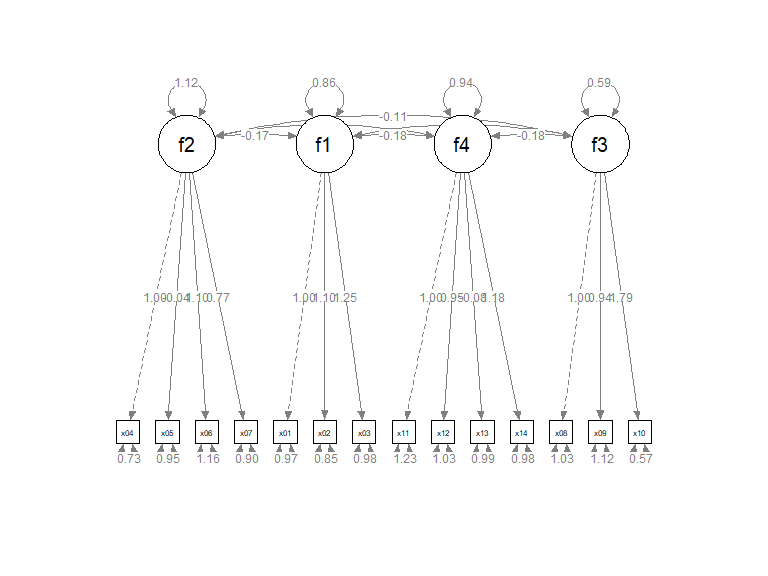# Change the Curvatures of the Factor Covariances

The graph has the factors and indicators ordered as required. However, the inter-factor covariances are too close to the factors. To increases the curvatures of the covariances, we can use the argument fcov_curve. The default is .4. Let us increase it to 1.75.

p2 <- set_cfa_layout(p,
indicator_order,
indicator_factor,
fcov_curve = 1.75)
plot(p2)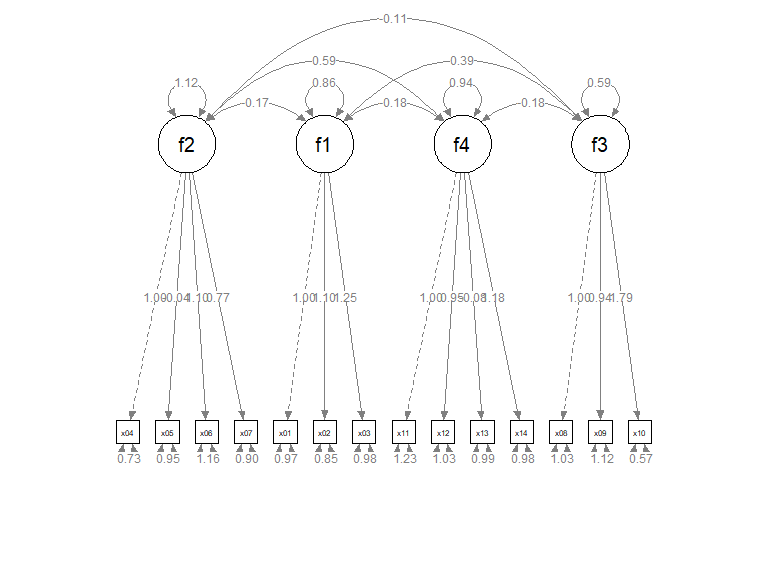The covariances are now more readable. The exact effect of the values vary from graph to graph. Therefore, trial and error is required to find a value suitable for a graph.

We can also move all the factor loadings together using the argument loading_position. The default value is .5, at the middle of the paths. If we want to move the loadings closer to the indicators, we increase this number. If we want to move the loadings closer to the indicators, we decrease this number. In the following example, we move the loadings closer to the indicators, and increase the distance between them in the process.

p2 <- set_cfa_layout(p,
indicator_order,
indicator_factor,
fcov_curve = 1.75,
plot(p2)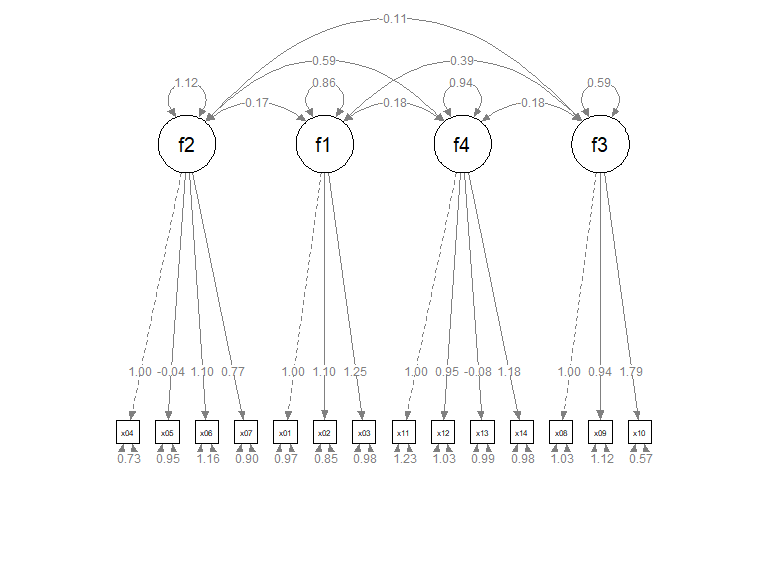# Rotate the Model

The default orientation is “pointing downwards”: latent factors on the top, pointing down to the indicators on the bottom. The orientation can be set to one of these four directions: down (default), left, up, and right. This is done by the argument point_to.

p2 <- set_cfa_layout(p,
indicator_order,
indicator_factor,
fcov_curve = 1.75,
point_to = "up")
plot(p2)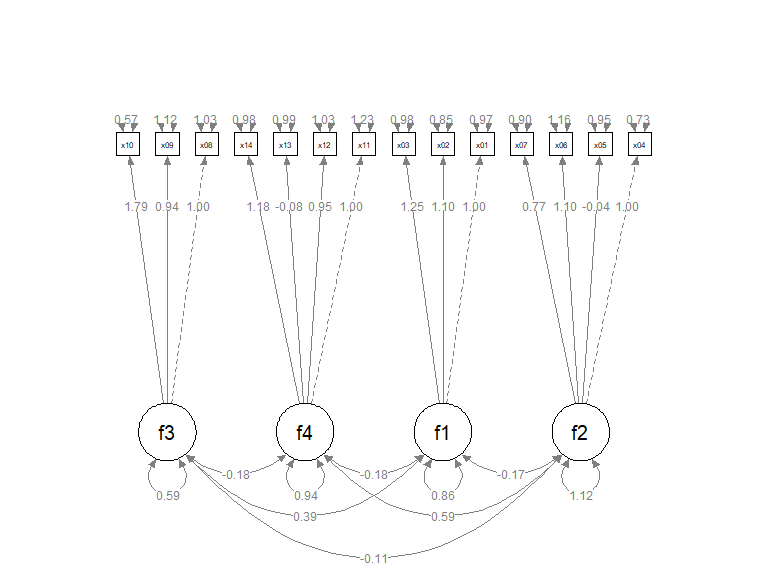# Pipe

Like other functions in semptools, the set_cfa_layout() function can be chained with other functions using the pipe operator, %>%, from the package magrittr, or the native pipe operator |> available since R 4.1.x. Suppose we want to mark the significant test results for the free parameters using mark_sig():

# If R version >= 4.1.0
p2 <- set_cfa_layout(p,
indicator_order,
indicator_factor,
fcov_curve = 1.75,
plot(p2)
#> Loading required package: magrittr